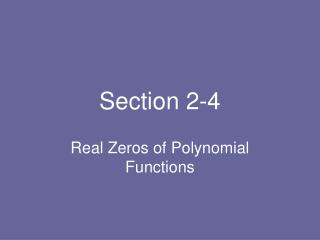DownloadDownload PresentationSection 2-4

# Section 2-4

Télécharger la présentation## Section 2-4

- - - - - - - - - - - - - - - - - - - - - - - - - - - E N D - - - - - - - - - - - - - - - - - - - - - - - - - - -
##### Presentation Transcript

1. Section 2-4 Real Zeros of Polynomial Functions

2. Section 2-4 • long division and the division algorithm • the remainder and factor theorems • reviewing the fundamental connection for polynomial functions • synthetic division • rational zeros theorem • upper and lower bounds

3. Long Division • long division for polynomials is just like long division for numbers • it involves a dividend divided by a divisor to obtain a quotient and a remainder • the dividend is the numerator of a fraction and the divisor is the denominator

4. Division Algorithm • if f(x) is the dividend, d(x) is the divisor, q(x) the quotient, and r(x) the remainder, then the division algorithm can be stated two ways

5. Remainder Theorem • if a polynomial f (x) is divided by x – k, then the remainder is f (k) • in other words, the remainder of the division problem would be the same value as plugging in k into the f (x) • we can find the remainders without having to do long division • later, we will find f (k) values without having to plug k into the function using a shortcut for long division

6. Factor Theorem • the useful aspect of the remainder theorem is what happens when the remainder is 0 • since the remainder is 0, f (k) = 0 which means that k is a zero of the polynomial • it also means that x – k is a factor of the polynomial • if we could find out what values yield remainders of 0 then we can find factors of polynomials of higher degree

7. Fundamental Connection For a real number k and a polynomial function f (x), the following statements are equivalent • k is a solution (or root) of the equation f (x) = 0 • k is a zero of the function f (x) • k is an x-intercept of the graph of f (x) • x – k is a factor of f (x)

8. Synthetic Division • finding zeros and factors of polynomials would be simple if we had some easy way to find out which values would produce a remainder of 0 (long division takes too long) • synthetic division is just that shortcut • it allows us to quickly divide a function f (x) by a divisor x – k to see if it yields a remainder of 0

9. Synthetic Division • it follows the same steps as long division without having to write out the variables and other notation • it is really fast and easy • if a zero is found, the resulting quotient is also a factor, and it is called the depressed equation because it will be one degree less than the original function

10. Synthetic Division

11. Synthetic Division 3 2 - 3 - 5 - 12

12. Synthetic Division 3 2 - 3 - 5 - 12 6 9 12 2 3 4 0

13. Synthetic Division 3 2 - 3 - 5 - 12 6 9 12 2 3 4 0 The remainder is 0 so x – 3 is a factor and the quotient, 2x2 + 3x + 4, is also a factor

14. Rational Zeros Theorems • if you want to find zeros, you need to have an idea about which values to test in S.D. (synthetic division) • the rational zeros theorem provides a list of possible rational zeros to test in S.D. • they will be a value where, p must be a factor of the constant q must be a factor of the leading term

15. Finding Possible Rational Zeros

16. Upper and Lower Bounds • a number k is an upper bound if there are no zeros greater than k; if k is plugged into S.D., the bottom line will have no sign changes • a number k is a lower bound if there are no zeros less than k; if k is plugged into S.D., the bottom line will have alternating signs (0 can be considered + or -)

17. Upper and Lower Bounds • if you are looking for zeros and you come across a lower bound, do not try any numbers less than that number • if you are trying to find zeros and you come across an upper bound, do not try any numbers greater than that number